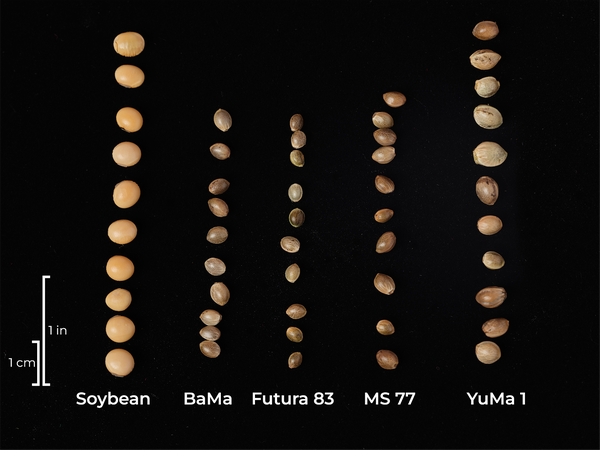NC State Extension Publications

## Not All Hemp Seed Are Created Equal

Whether you’re planting hemp for fiber, grain, or dual-purpose, having the appropriate plant populations is critical. A fiber-specific crop will require a very high seeding rate (over 1 million seed/ac) versus a grain-specific crop (400,000 – 500,000 seed/ac). Farmers often like to think in terms of seed weight per acre, for example, 50 lb/ac, and many seeding rate recommendations will be made in pounds per acre. However, there is significant variability in grain size among the diverse fiber and grain hemp varieties. Planting based on seed weight without accounting for grain size may result in over-or under-planting and not meeting the crop population target.

Ideally, the seed provider should supply 1,000 kernel weight or the number of seed per pound. If not, a sample of at least 200 seeds should be weighed to generate an individual seed weight. The seed provider should also provide a germination rate. These two numbers will allow you to accurately calculate the weight of seed needed to meet your desired population.

To demonstrate, let’s compare four fiber-specific hemp varieties: BaMa, Futura 83, MS 77, and YuMa 1 (Figure 1)

We weighed 1,000 seeds to obtain the 1,000 kernel weight (Table 1). One hundred seeds were placed in a wet paper towel, placed in a plastic bag, and left in a warm, dark location for five days to calculate the germination rate.Figure 1. Seed size variability among four fiber hemp varieties compared to soybean.

David Suchoff

Table 1. Fiber hemp seed germination rates and weights

Variety 1,000 kernel weight
(g)
Germination rate
(%)
BaMa 27 70
Futura 83 20 88
MS 77 28 84
YuMa 1 42 91

To calculate the amount of seed necessary to have 1 million plants/ac we first determine the weight of 1 million seed:

BaMa: $$\left(\frac{27\ g}{1,000\ seed}\right)=\left(\frac{x}{1,000,000\ seed}\right); x=27,000\ g\ \text{or}\ 27\ kg$$

Futura 83: $$\left(\frac{20\ g}{1,000\ seed}\right)=\left(\frac{x}{1,000,000\ seed}\right); x=\ 20,000\ g\ \text{or}\ 20\ kg$$

MS 77: $$\left(\frac{28\ g}{1,000\ seed}\right)=\left(\frac{x}{1,000,000\ seed}\right); x=\ 28,000\ g\ \text{or}\ 28\ kg$$

YuMa 1:$$\left(\frac{42\ g}{1,000\ seed}\right)=\left(\frac{x}{1,000,000\ seed}\right); x=\ 42,000\ g\ \text{or}\ 42\ kg$$

Next, we need to take into account the germination rate to calculate the amount of seed required for 1,000,000 pure live seed (PLS):

BaMa: $$\left(\frac{27\ kg}{1,000,000\ seed}\right)\div\ 0.70\ =\ 38.67\ kg\$$

Futura 83: $$\left(\frac{20\ kg}{1,000,000\ seed}\right)\div\ 0.88\ =\ 22.73\ kg\$$

MS 77: $$\left(\frac{28\ kg}{1,000,000\ seed}\right)\div\ 0.84=\ 33.33\ kg\$$

YuMa 1: $$\left(\frac{42\ kg}{1,000,000\ seed}\right)\div\ 0.91\ =\ 46.15\ kg\$$

Finally, we convert to pounds to give the weight required to plant 1,000,000 PLS/ac.

BaMa: $$38.67\;kg\;\times\;2.20462\;{\frac{lb}{kg}} = 85.25\;lb$$

Future 83: $$22.73\;kg \;\times\; 2.20462\;{\frac{lb}{kg}} = 50.11\;lb$$

MS 77: $$33.33\;kg \;\times\; 2.20462\;{\frac{lb}{kg}} = 73.48\;lb$$

YuMa 1: $$46.15 \;\times\; 2.20462\;{\frac{lb}{kg}} = 101.74\; lb$$

When taking seed weight and germination rate into account we see that the amount of seed required to plant 1,000,000 PLS varies considerably!

Now that we have our required seed weight, the last step is to calibrate our grain drill to ensure we are planting seed at the appropriate rate. The following video demonstrates the appropriate steps to calibrating your small grain drill.

# Authors

Alternative Crops Extension Specialist
Crop and Soil Sciences
Alternative Crops Research Technician
Crop and Soil Sciences